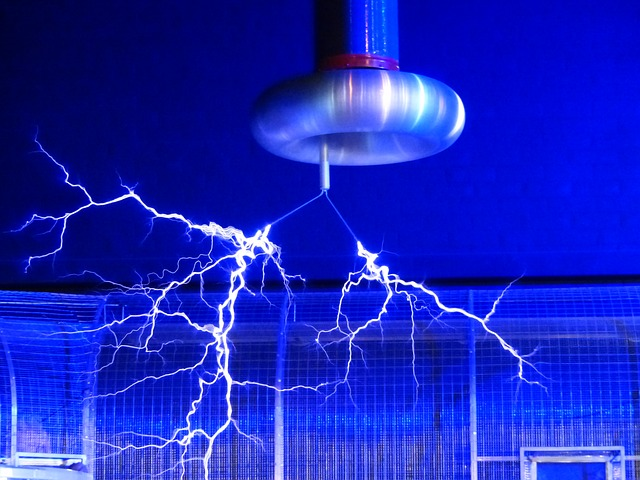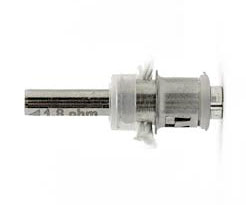# Basic Electricity for BeginnersOne thing that e cig beginners have said to me repeatedly is that they don’t understand what ohms and volts and watts are and why it’s useful to know about them.If you’re really not interested, the best thing you can do is to buy a regulated setup that comes complete with battery and atomiser. It’s only when you start to use separate batteries, mods & atomisers that this stuff starts to be important and sadly, most of the ‘exploding e-cigs’ stories come down to not understanding this stuff or ignoring battery safety. So, if this really isn’t your thang, then please only use the charger, batteries & tank that came with your device. When charging batteries, never leave them unattended, take them off charge as soon as they are charged and only carry batteries in a battery box . If you are interested in finding out a little more, read on.

### Ohm’s LawThere are plenty of websites out there where you can learn about the physics & history behind all this if you are interested. As a vaper, you only really need to understand the principles and the relationship. A commonly used analogy is that of a water system. It’s not perfect but it seems to help most people.

### Pressure = Voltage

In a water system you can measure water pressure as the height of the column of water that it would produce. In an electrical system pressure is measured in volts. A typical e-cig battery has a voltage of 3.7 volts. Electronic circuits are used to either stabilise this level or to increase it to a higher level.

### Flow = Current

Cubic feet per second can be used to measure the flow rate of water. The electrical equivalent is Amperes. This is often shortened to ‘amps’ or just ‘A’. One thousandth of an amp is a milliamp (mA).

### Capacity or flow over time = milliAmpere hours

With e-cig batteries you’ll see the capacity stated in milliAmpere hours (mAh). For example, a 2000 mAh battery should be able to deliver 2 amps for one hour, one amp for two hours or 200mA for 10 hours.

### Restriction = Resistance

In a water system, where there is a restriction in a pipe, the flow of water is impeded. The electrical equivalent of this is resistance and it’s measured in ohms. In an atomiser, the coil is often made from special alloys, such as iron, chromium and aluminium. These produce heat because of their resistance.

### Resistance and Ohm’s law

In the picture below, you can see the relationship between current flow & resistance by moving the slider to change resistance in the circuit. With the slider at the right, its resistance is zero and when its at the left resistance is infinity.

As the resistance of the slider decreases, the flow of current increases & the lamp begins to light-up. As the slider resistance increases, the flow of current becomes lower & the lamp dims. In this example, the voltage has remained constant and changing the resistance changed the current.

In fact, there is a simple equation that describes the relationship:

Voltage = Current X Resistance and this is usually written as V = I x RIn an e-cig, the resistance is more or less fixed (resistance does actually change a little as the coil heats), so we need to rearrange the formula to make it more use to us as vapers. So re-arranging it, we get:

Resistance = Voltage divided by Current or R = V/I

and

Current = Voltage divided by Resistance or I = V/R

So, if we know the voltage and the resistance, we can calculate the current and if we know the voltage and current, we can calculate the resistance.Suppose our atomiser has a resistance of 2 ohms (this is often marked as 2Ω) and our battery has a voltage of 3.6 volts. We can calculate the current flowing:

3.6/2 = 1.8 Amps

If our atomiser had a resistance of 1Ω and the voltage was 3.6 volts, our current would be:

3.6/1 = 3.6 Amps

### PowerWe’ve seen the relationship between voltage, current and resistance above but there is another factor that vapers need to understand. That is power. It is measured in watts and is the product of current and voltage.

Power = Voltage x Current and is usually written as P = I x V

In the picture below, you can alter both the voltage delivered to the circuit and the resistance. Moving the voltage slider to the right increases voltage and moving the resistance slider to the right increases resistance. You can see the resulting power consumed in watts at the top left.

The power equation (P = I x V) can be re-arranged just like the one for Ohm’s law, so we can derive these formulas from it:

Current = Power divided by Voltage (I = P/V) and

Voltage = Power divided by Current (V = P/I)

Using the same example as above, where our atomiser had a resistance of 2 ohms and our battery had a voltage of 3.6 volts, we calculated the current flowing to be 1.8 Amps.

Now we can calculate the power by using the new formula:

P = I x V   or P = 1.8 x 3.6 = 6.48 Watts

### Pulling it all together

The two simple equations enable us to solve for each of the components involved and we can also combine them together to produce some more useful formulas. The original equations and those that are derived are shown below:

P = V² x R         I = P/V              R = V²/P        V = √(P x R)       V = I x R        R = V/I       I = V/R

P = I² x R          I = √P/R           R = P/I²         V = P/I                 P = I x V         I = P/V

### How is this useful?To be fair, understanding this sort of thing isn’t really important until you get beyond basic e-cigs. However, anyone going ‘sub-ohm’ really should have a good grasp of what they’re doing. I saw a post on a forum some time back where someone was saying that they’d built a coil with a resistance of 0.01 ohms and they were intending to use it in a single battery mechanical mod. Now, that’s all well and good but a few quick calculations highlight the dangers:

R = 0.01Ω

Lets say voltage = 3.7 Volts & we can calculate the current:

I = V/R   so I = 3.7/0.01 = 370 Amps and Power = V x I = 3.7 x 370 = 1369 Watts

That might just lead to a hot vape for a few seconds before the battery goes into thermal runway and then explodes or hopefully, the coil or wiring disintegrates first & it stops working.

Being able to do calculations like these enables you to ensure that the battery that you’re intending to use is actually capable of delivering the current that the atomiser will draw.

I usually use a variable wattage device but if I had to use a mod that was only able to do variable voltage, I could easily and quickly set my desired power by knowing the atomiser resistance and doing a quick calculation. For example, if my atomiser was 1.8Ω and I wanted to vape at 15 Watts, I could  easily calculate the voltage using the formula above:

V = √(P x R)  so Voltage = √(15 x 1.8) = 5.196 Volts  and 5.2 Volts is near enough for rock n roll

Originally Published by Dave Upton 2015 – Reproduced by ocdz.co.uk with kind permission

###### 1 Pings/Trackbacks for "Basic Electricity for Beginners"
1. […] Basic Electricity for Beginners […]